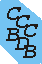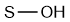Computational Chemistry Comparison and Benchmark DataBase Release 22 (May 2022) Standard Reference Database 101 National Institute of Standards and Technology Home All data for one species Geometry Experimental Calculated Comparisons Bad Calculations Tutorials and Explanations Vibrations Experimental Calculated Scale factors Reactions Entropies Ions List Ions Energy Electron Affinity Proton Affinity Ionization changes point group Experimental One molecule all properties One property a few molecules Geometry Vibrations Energy Electrostatics Reference Data Calculated Energy Optimized Reaction Internal Rotation Orbital Nuclear repulsion energy Correlation Ion Excited State Basis Set Extrapolation Geometry Vibrations Frequencies Zero point energy (ZPE) Scale Factors Bad Calculations Electrostatics Charges Dipole Quadrupole Polarizability Spin Entropy and Heat Capacity Reaction Lookup by property Comparisons Geometry Vibrations Energy Entropy Electrostatics Ion Resources Info on Results Calculations Done Basis functions used I/O files Glossary Conversion Forms Links NIST Links External links Thermochemistry Tutorials Vibrations Entropy Energy Electrostatics Geometry Cost Bad Calculations FAQ Help Units Choose Units Explanations Credits Just show me Summary Using List Recent molecules Molecules Geometry Vibrations Energy Similar molecules Ions, Dipoles, etc. Index of CCCBDB Feedback You are here: Comparisons > Energy > Similar molecules > Isomers OR FAQ Help > List > Similar molecules > Isomers

# Relative enthalpies of isomers - Comparison of 298.15K enthalpies (kJ mol-1)

## Isomers of HOS

index Species CAS number Name Relative experimental enthalpy (kJ mol-1) sketch
a HSO+ 62470717 Sulfur Monoxide, S-protonatedb SOH+ 62803127 Sulfur Monoxide, protonatedThe calculated enthalpies include the calculated and scaled vibrational zero-point energy.
semi-empirical 878.4 a 801.3 b 873.0 a 830.0 b 821.4 a 759.7 b NCNC NCNC NCNC NCNC NCNC NCNC NCNC

NC = not calculated
For descriptions of the methods (AM1, HF, MP2, ...) and basis sets (3-21G, 3-21G*, 6-31G, ...) see the glossary in section I.C. Predefined means the basis set used is determined by the method.
gaw refers to the group additivity method implemeted in the NIST Chemistry Webbook.
See section Calculated; Vibrations; Scale Factors; Scale factors to list vibrational scaling factors.
See section Calculated; Vibrations; Scale Factors; Calculate a scale factor to calculate a vibrational scaling factor for a given set of molecules.